# Student Grade Calculator using Java Swing

Consider the following scenario. We need to create a GUI based application which calculates grade of students according to marks obtained in 4 subjects. Below are the range of marks for different grades.

We will be using Netbeans to create GUI applications since it’s a well crafted open-source IDE that provides features of a Component inspector, Drag-and-Drop of widgets, Debugger, Object browser, etc.

### Steps to create the Student Grade Calculator

1. Create a new Java application by clicking on New Project -> Java -> Java Application and give a suitable project name. Eg: GeeksForGeeks and click Finish.

2. To create a New File under the same Java project GeeksForGeeks, right-click on the project name on the left-hand side of the window, click New -> JFrame Form and give a suitable file name. Eg. Grade_Calulator.java and click Finish.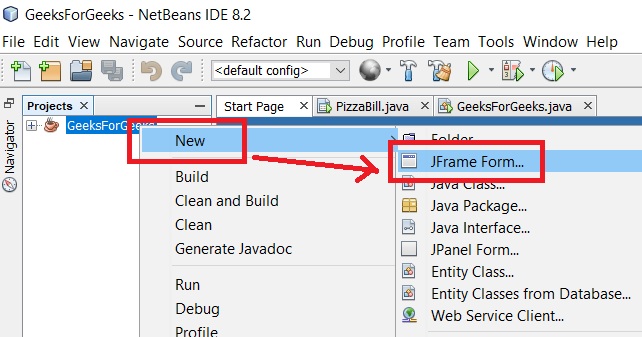Creating New File in existing Java Application Project

3. Now from the palette situated at the right-hand side of the window, start dragging the toolkit widgets as per requirements. To change the background color of the frame, we need to first insert a JPanel and change its properties.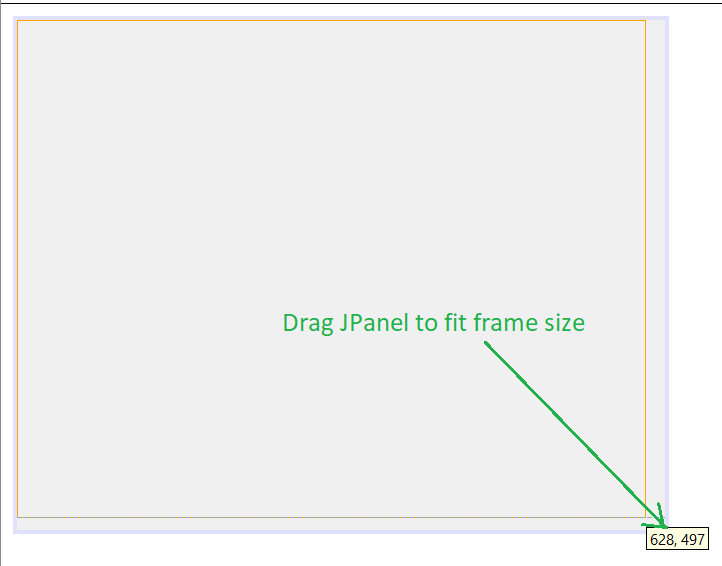Drag the panel to fit the frame size

4. Click anywhere on the panel area, go to properties -> background.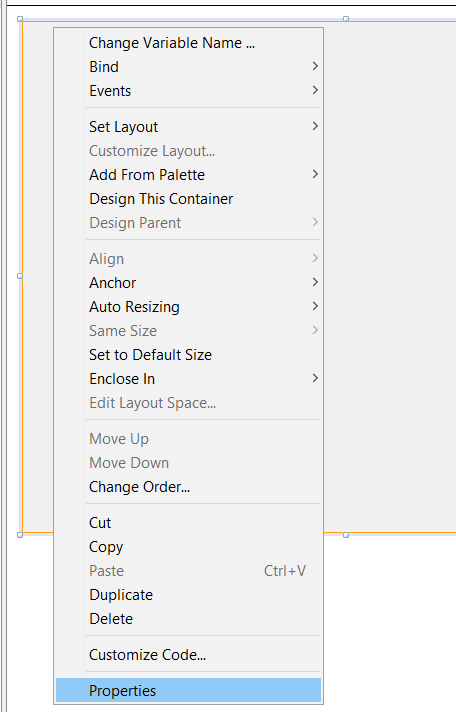5. Double click on the background option and select any color of your choice. Then click OK.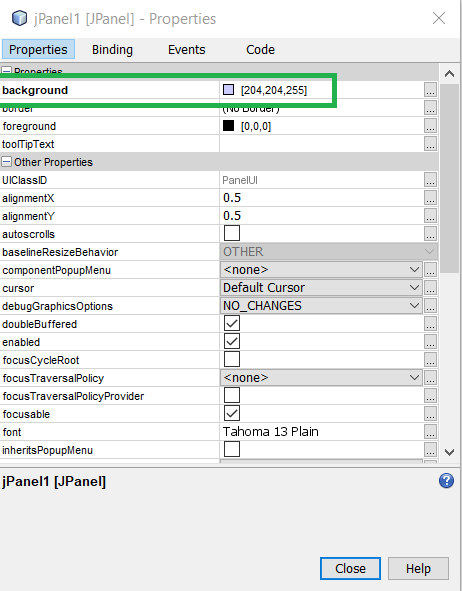6. After setting background color, drag other widgets onto the design area. To display student details like Student ID, Name, Grade in a separate Dialog Box, drag a JOptionPane widget from the palette. First it overlaps the existing design. Go to navigator on left-hand side of the screen, then drag and move the JOptionPane component from JFrame to Other Components. It will go behind.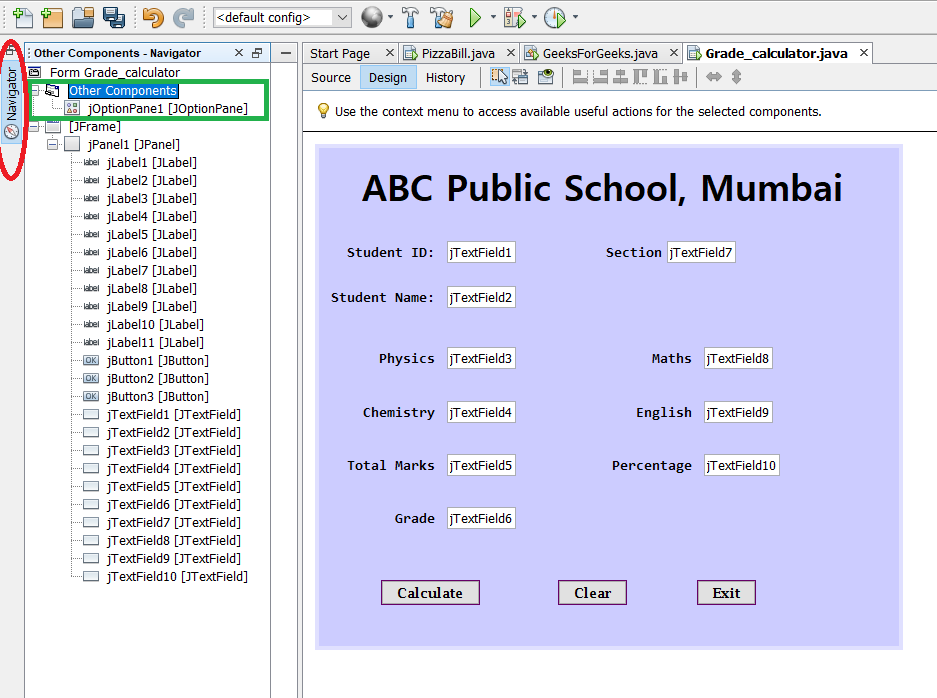7. Let us now quickly go through the usage of each component in the program tabulated below.

8. Now to type the code, double click on jButton1, you will be directed to the source tab. Here type in the following code.

## Java

 `// Initialize variables ` `int` `s_id = Integer.parseInt(jTextField1.getText()); ` `String s_name = jTextField2.getText(); ` `String s_sec = jTextField7.getText(); ` `double` `phy = Double.parseDouble(jTextField3.getText()); ` `double` `chem = Double.parseDouble(jTextField4.getText()); ` `double` `maths = Double.parseDouble(jTextField8.getText()); ` `double` `eng = Double.parseDouble(jTextField9.getText()); ` ` `  `// Calculates total marks ` `double` `total = phy + chem + maths + eng; ` `jTextField5.setText(``""` `+ total); ` ` `  `// Calculates percentage ` `double` `per = (total / ``400``) * ``100``; ` `jTextField10.setText(``""` `+ per); ` `String grade = ``null``; ` ` `  `// Grade calculation ` `if` `(per > ``90``) { ` `    ``grade = ``"A"``; ` `} ``else` `if` `((per > ``85``) && (per < ``90``)) { ` `    ``grade = ``"B"``; ` `} ``else` `if` `((per > ``80``) && (per < ``85``)) { ` `    ``grade = ``"C"``; ` `} ``else` `if` `((per > ``70``) && (per < ``80``)) { ` `    ``grade = ``"D"``; ` `} ``else` `if` `((per > ``60``) && (per < ``70``)) { ` `    ``grade = ``"E"``; ` `} ``else` `if` `((per > ``50``) && (per < ``60``)) { ` `    ``grade = ``"Poor"``; ` `} ` ` `  `// Displays result in TextField ` `jTextField6.setText(``""` `+ grade); ` ` `  `// Displays result in new Dialog Box ` `jOptionPane1.showMessageDialog(``null``, ``"Hello: "` `+ s_name + ``" of class: "` `+ s_sec + ` `    ``"\nYour Grade is: "` `+ grade); `

9. Now to clear all textfields write the following code under the jButton2 ActionPerformed option which can be achieved by clicking twice on Clear Button in the design area.

## Java

 `jTextField1.setText(``""``); ` `jTextField2.setText(``""``); ` `jTextField3.setText(``""``); ` `jTextField4.setText(``""``); ` `jTextField5.setText(``""``); ` `jTextField6.setText(``""``); ` `jTextField7.setText(``""``); ` `jTextField8.setText(``""``); ` `jTextField9.setText(``""``); ` `jTextField10.setText(``""``);`

10. Now to exit from the system, use the following statement under the jButton3 ActionPerformed option which can be achieved by clicking twice on exit button in the design area.

``` System.exit(0)

```

11. Now, right-click anywhere on the screen and select Run File option from the drop-down menu. The final output is shown below. Input necessary details and the application is ready!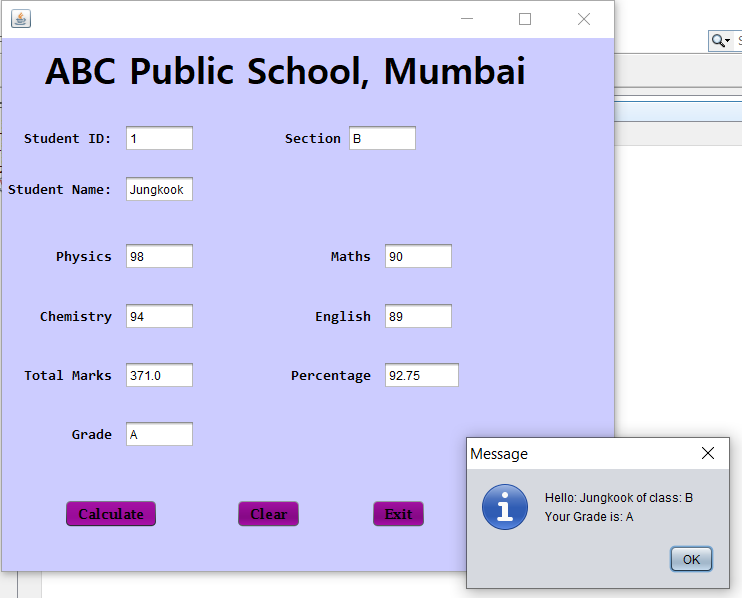Final Output

Output:

Attention reader! Don’t stop learning now. Get hold of all the important Java and Collections concepts with the Fundamentals of Java and Java Collections Course at a student-friendly price and become industry ready.

My Personal Notes arrow_drop_upCheck out this Author's contributed articles.

If you like GeeksforGeeks and would like to contribute, you can also write an article using contribute.geeksforgeeks.org or mail your article to contribute@geeksforgeeks.org. See your article appearing on the GeeksforGeeks main page and help other Geeks.

Please Improve this article if you find anything incorrect by clicking on the "Improve Article" button below.

Article Tags :
Practice Tags :

Be the First to upvote.

Please write to us at contribute@geeksforgeeks.org to report any issue with the above content.